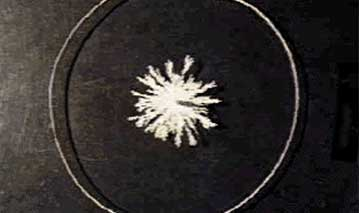4.1 - Growing Rough Patterns: ElectrodepositionHandsOn Activities:

13. Growing a Pattern in the Laboratory

Is a coastline a line? Not really, because a line is one-dimensional, whereas a coastline is a random fractal that has a dimension whose value is between 1 and 2. Other random fractal patterns also have dimensions between 1 and 2: a snowflake, a nerve cell, a lightning stroke. The growth of these structures can be modeled by a process called aggregation, in which random walkers dance around the growing structure and stick to it when they touch it. The resulting jagged pattern is called an aggregate. Depending on the details of the growth process, some aggregates are natural fractals, others are not.

The shiny chromium surfaces on an expensive automobile are made by immersing the parts into a chemical bath that contains chromium ions and running an electric current through the bath so that chromium metal attaches to the parts to be coated. This process is called electroplating or electrodeposition.

The goal in chromium electroplating is to produce a smooth surface so the car will look good and be protected against rust. In our pattern-forming experiment, the result will be quite different from this, as you will see.

To understand the experiment, you need to recall that:

(a) In the electrochemical deposition (ECD) experiment, a copper ion, for example, gains two electrons at the negative terminal and precipitates out as a copper atom, so that the negative terminal grows.

(b) Avogadro's number is 6.02  . 1023 molecules = 1 mole of molecules.

(c) The concentration of a solution is measured in molarity (symbol M). The molarity refers to how many moles of solute there are in a liter of solution. For the purposes of these experiments, we will not take into account volume expansion when the solute is added to water. So, we will interpret 0.1 M Cu2SO4 (aq) as being 0.1 mole of Cu2SO4 in 1 liter of water. (The abbreviation "aq'' means "in aqueous solution.'' The Latin word "aqua'' means "water'' and is the root of the word "aquarium.'')

(d) A coulomb is a measure of electric charge equal to 6.24   . 1018 elementary charges (the negative charge on the electron and the positive charge on the proton). Current is the flow of charge; an ampere is a measure of current: 1 ampere = 1 coulomb/second.

(e) It will help your reasoning during the experiment to remember the relationship between voltage V and electric field E. If a voltage V is applied between two electrodes, an electric field is set up that points away from the positive electrode, and towards the negative electrode. A positive charge moves in the direction of the electric field. For the geometry of the cell used in this experiment (a vertical-wire negative terminal circled by a positive terminal) the electric field is strongest near the central wire.

(f) When thinking about the flow of ions in the electrodeposition cell, take into account the resistance of the salt solution. Recall that the resistance R in ohms of an object of cross-section A, length L, and resistivity r is: R = rL/A.

(g) The applied voltage V, resistance of the circuit R, and the current that flows I, are related by the equation V = I R. For example, if the applied voltage across your cell (i.e., between the positive terminal and negative terminal) is 10 volts, and the resistance of the cell 100 ohms, then the current that flows through the cell is 0.1 ampere or 100 milliamperes.

Previous: Unit 4 - Physical and Chemical Branching Patterns